• 一个无穷级数展开式@(微积分)诸如∑+∞i=0xi\sum_{i=0}^{+\infty}x^i是非常常见的无穷级数展开式运用。还有变形：∑+∞i=1xi\sum_{i=1}^{+\infty}x^i等等有细微的不同。但根子都在一个展开式上：11−x=1+x+x2+...+xn...
一个无穷级数展开式

@(微积分)

诸如∑+∞i=0xi<!--//--><![CDATA[//><!--
\sum_{i=0}^{+\infty}x^i
//--><!]]>是非常常见的无穷级数展开式运用。还有变形：∑+∞i=1xi<!--//--><![CDATA[//><!--
\sum_{i=1}^{+\infty}x^i
//--><!]]>等等有细微的不同。但根子都在一个展开式上：

11−x=1+x+x2+...+xn,x∈(−1,1)<!--//--><![CDATA[//><!--
{1\over 1-x} = 1+x+x^2+...+x^n, x\in (-1,1)
//--><!]]>

所以，直接可以得到的是：

∑i=0+∞xi=11−x<!--//--><![CDATA[//><!--
\sum_{i=0}^{+\infty}x^i = {1\over 1-x}
//--><!]]>稍加变形：

∑i=1+∞xi=x∑i=0+∞xi=x1−x<!--//--><![CDATA[//><!--
\sum_{i=1}^{+\infty}x^i = x\sum_{i=0}^{+\infty}x^i = {x\over 1-x}
//--><!]]>特别注意下标的起始，是非常关键的点。

还可以求∑+∞i=1ixi<!--//--><![CDATA[//><!--
\sum_{i=1}^{+\infty}ix^i
//--><!]]>

∑i=1+∞ixi=x∑i=1+∞ixi−1=x∑i=1+∞(xi)′=x(∑i=1+∞xi)′=x(x1−x)′<!--//--><![CDATA[//><!--
\sum_{i=1}^{+\infty}ix^i  = x \sum_{i=1}^{+\infty}ix^{i-1} = x\sum_{i=1}^{+\infty}(x^i)\prime \\
= x(\sum_{i=1}^{+\infty}x^i)\prime \\
= x({x\over 1-x})\prime
//--><!]]>举个在概率中化用的例子：
设随机变量X取非负整数值，P(X=n)=an(n≥1),EX=1<!--//--><![CDATA[//><!--
P(X=n)=a^n(n\geq 1),EX = 1
//--><!]]>,则a=？<!--//--><![CDATA[//><!--
a = ？
//--><!]]>

求解：

EX=a+2a2+3a3+...=∑+∞i=1iai=1<!--//--><![CDATA[//><!--
EX = a+2a^2+3a^3+... = \sum_{i=1}^{+\infty}ia^i = 1
//--><!]]>

如果熟练使用无穷级数工具，则问题会很简单。

EX=a∑i=1+∞iai−1=a(∑i=1+∞ai)′=a(a1−a)′=a(1−a)2=1<!--//--><![CDATA[//><!--
EX = a\sum_{i=1}^{+\infty}ia^{i-1} = a(\sum_{i=1}^{+\infty}a^{i})\prime \\
= a({a\over 1-a})\prime = {a\over(1-a)^2} = 1
//--><!]]>由此可解出

a=3−5√2<!--//--><![CDATA[//><!--
a = {3-\sqrt 5\over 2}
//--><!]]>
展开全文微积分
• 几种常见波形的傅里叶级数展开式 梯形波（奇函数） 傅里叶展开为： 脉冲波（偶函数） 傅里叶展开为： 方波（奇函数） 傅里叶展开为： 三角波（奇函数） 傅里叶展开为： 锯齿波（非奇非偶函数） 傅里叶...

公式
给定一个周期为的函数，那么它可以表示为无穷级数：其中傅里叶系数为：几种常见波形的傅里叶级数展开式

梯形波（奇函数）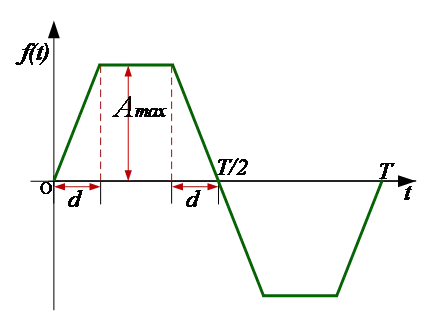傅里叶展开为：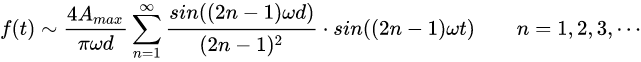脉冲波（偶函数）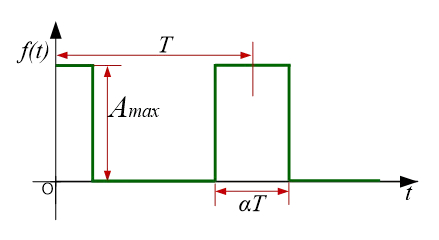傅里叶展开为：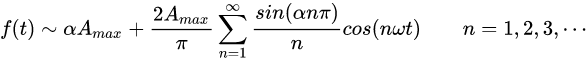方波（奇函数）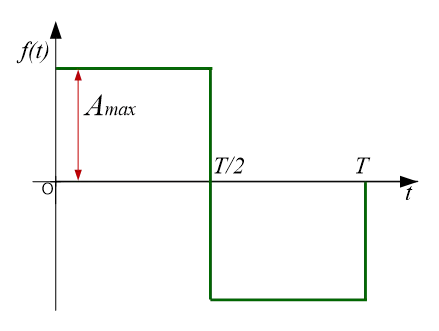傅里叶展开为：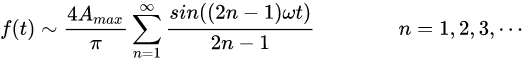三角波（奇函数）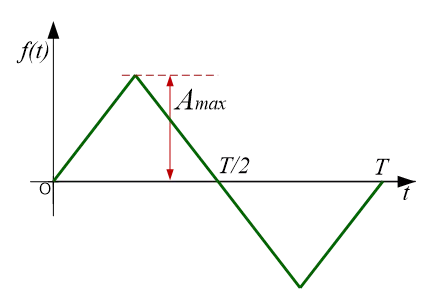傅里叶展开为：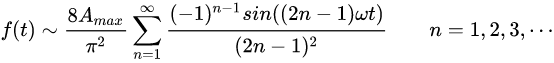锯齿波（非奇非偶函数）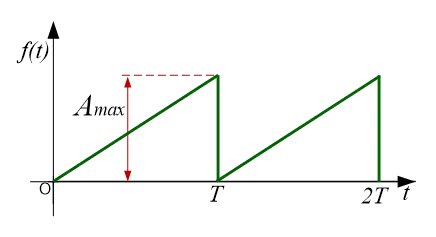傅里叶级数展开式为：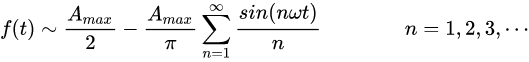展开全文线性代数 矩阵
• 因为日常计算中经常需要做一些近似，而泰勒级数展开是其中最常用的一种，所以本篇整理了部分常见的（一元函数）泰勒公式展开
因为日常计算中经常需要做一些近似，而泰勒级数展开是其中最常用的一种，所以本篇整理了部分常见的（一元函数）泰勒公式展开

常见泰勒级数展开一、泰勒中值定理二、常用的泰勒级数展开

一、泰勒中值定理
对于简单的多项式函数，我们往往是很喜欢的，但是我们要接触的往往是一些比较复杂的函数，所以一种自然而然的想法也就出来了，那就是利用多项式来近似逼近这些复杂的函数，这就不得不提我们非常熟悉的泰勒展开了，在具体列出常见的泰勒展开之前，我觉得还是有必要提一嘴泰勒中值定理的（好吧，其实是为了凑字数），下面就介绍一下泰勒中值定理。

泰勒（Taylor）中值定理（一元函数）

如果函数$f(x)$在含有$x_0$的某个开区间$(a,b)$内具有知道$(n+1)$阶的导数，则对任一$x\in(a,b)$，有
$f(x)=f(x_0)+f'(x_0)(x-x_0)+\frac{f''(x_0)}{2!}(x-x_0)^2+\cdots+\frac{f^{(n)}(x_0)}{n!}(x-x_0)^n+R_n(x)$其中
$R_n(x)=\frac{f^{(n+1)}(\xi)}{(n+1)!}(x-x_0)^{n+1}$这里的$\xi$是$x_0$与$x$之间的某个值。
这个定理则告诉我们，如果已知某个函数在某一点的$n+1$阶导数，那么我们原则上是可以将这个函数在这个点附近用一个多项式进行逼近的，但是上面的这个定理其实是基于一元函数的，为了方便以后的扩展，下面在简单介绍一下多元函数的泰勒中值定理。

多元函数的泰勒中值定理

设$f=f(x^1,x^2,\cdots,x^k)$在点$(x^1_0,x^2_0,\cdots,x^k_0)$的某一领域内连续且有直到$n+1$阶的连续偏导数,$\\(x^1_0+h_1,x^2_0+h_2,\cdots,x^k_0+h_k)$为此邻域内的任一点，则有
$f(x^1_0+h_1,x^2_0+h_2,\cdots,x^k_0+h_k) = f(x^1_0,x^2_0,\cdots,x^k_0)$
$+\sum_{i=1,2,\cdots,k}\frac{\partial{f(x^1_0,x^2_0,\cdots,x^k_0)}}{\partial{x^i}}h_i+\frac{1}{2!}\sum_{i_1=1}^{k}\sum_{ i_2=1}^{k}\frac{\partial^2f(x^1_0,x^2_0,\cdots,x^k_0)}{\partial{x^{i_1}}\partial{x^{i_2}}}h_{i_1}h_{i_2}+$
$\cdots+\frac{1}{n!}\sum_{i_1=1}^{k}\sum_{i_2=1}^{k}\cdots\sum_{i_n=1}^{k}\frac{\partial^nf(x^1_0,x^2_0,\cdots,x^k_0)}{\partial{x^{i_1}}\partial{x^{i_2}}\cdots\partial{x^{i_n}}}\prod_{j={i_1}}^{i_n}h_j+R_n(x^1_0,x^2_0,\cdots,x^k_0)$
其中
$R_n(x^1_0,x^2_0,\cdots,x^k_0)=\frac{1}{(n+1)!}\sum_{i_1=1}^{k}\sum_{i_2=1}^{k}\cdots\sum_{i_{n+1}=1}^{k}\frac{\partial^nf(x^1_0+\theta h_1,x^2_0+\theta h_2,\cdots,x^k_0+\theta h_k)}{\partial{x^{i_1}}\partial{x^{i_2}}\cdots\partial{x^{i_n}}}\prod_{j={i_1}}^{i_{n+1}}h_j ,(0<\theta<1)$
二、常用的泰勒级数展开
基于上面的泰勒中值定理，可以导出一些常见函数的泰勒展开公式（具体推导不做展开，具体可参见同济版高数上册），然后由泰勒展开公式结合无穷级数可以得到下面的幂级数展开式：
$e^x=\sum_{n=0}^\infty \frac{x^n}{n!}=1+x+\frac{x^2}{2!}+\cdots+\frac{x^n}{n!}+\cdots,-\infty
$\frac{1}{1+x}=\sum_{n=0}^\infty(-1)^nx^n=1-x+x^2-\cdots+(-1)^nx^n+\cdots,-1
$ln(1+x)=\sum_{n=1}^\infty(-1)^{n-1}\frac{x^n}{n}=x-\frac{x^2}{2}+\frac{x^3}{3}-\frac{x^4}{4}+\cdots+(-1)^{n-1}\frac{x^n}{n}+\cdots,-1
$sinx=\sum_{n=0}^\infty(-1)^n\frac{x^{2n+1}}{(2n+1)!}=x-\frac{x^3}{3!}+\frac{x^5}{5!}-\frac{x^7}{7!}+\cdots+\frac{x^{2n+1}}{(2n+1)!}+\cdots,-\infty
$cosx=\sum_{n=0}^\infty(-1)^n\frac{x^{2n}}{(2n)!}=1-\frac{x^2}{2!}+\frac{x^4}{4!}-\frac{x^6}{6!}+\cdots+(-1)^n\frac{x^{2n}}{(2n)!}+\cdots,-\infty
$(1+x)^\alpha=1+\alpha x+\frac{\alpha (\alpha-1)}{2!}x^2+\cdots+\frac{\alpha (\alpha-1)\cdots (\alpha-n+1)}{n!}x^n+\cdots,\begin{cases}x\in(-1,1),\alpha\leqslant-1 \\ x\in(-1,1],-1<\alpha<0 \\ x\in[-1,1],\alpha>0\end{cases}$

同济版高数


展开全文数学
• 近来，在开展课题时遇到了需要将梯形波进行傅里叶级数展开的问题，查询了一些资料（惭愧，一开始就没想着自己动手积分），然后没有找到自己想要的结果（其实有相近的，只不过不是任意周期的，当时没有转变过来），...
引言
近来，在开展课题时遇到了需要将梯形波进行傅里叶级数展开的问题，查询了一些资料（惭愧，一开始就没想着自己动手积分），然后没有找到自己想要的结果（其实有相近的，只不过不是任意周期的，当时没有转变过来），最后还是动手算出来了，在这里做一个小小的记录，算是回顾以前的知识吧，捂脸。
由于像三角波，矩形波，梯形波这种波形不连续，因此在仿真软件中很容易出现计算不收敛的情况。所以，在这种情况下，利用一系列谐波叠加的形式来等价于原来的波形，可以很好的优化模型。

预备知识
1. 公式
给定一个周期为的函数，那么它可以表示为无穷级数：其中傅里叶系数为：2. 性质
收敛性
在闭区间上满足狄利克雷条件的函数表示成的傅里叶级数都收敛。狄利克雷条件如下：

在定义区间上，需绝对可积；
在任一有限区间中，只能取有限个极值点；
在任何有限区间上，只能有有限个第一类间断点。

满足上述条件的傅里叶级数都收敛，且：

当是的连续点时，级数收敛于当是的间断点时，级数收敛于正交性
所谓的两个不同向量正交是指它们的内积为0，这也就意味着这两个向量之间没有任何相关性，例如，在三维欧式空间中，互相垂直的向量之间是正交的。三角函数族的正交性用公式表示出来就是：奇偶性
奇函数可以表示为正弦级数，而偶函数则可以表示成余弦级数：几种常见波形的傅里叶级数展开式
1. 梯形波（奇函数）
梯形波
如上图所示，该梯形波是一个周期为T的奇函数，幅值为，上升沿时间为，在区间的函数表达式为：由奇偶性可知，该波形在区间的傅里叶级数展开式为：其中傅里叶系数为：将函数代入傅里叶系数表达式中，可得：由可得：综上所述，可以得到该梯形波在区间的傅里叶级数展开式为：其中：2. 脉冲波（偶函数）
脉冲波
如上图所示，该脉冲波是一个周期为T的偶函数，幅值为，脉冲宽度为，在区间的函数表达式为：由奇偶性可知，该波形在区间的傅里叶级数展开式为：其中傅里叶系数为：将函数代入傅里叶系数表达式中，可得：因此，可以得到该梯形波在区间的傅里叶级数展开式为：其中：3. 方波（奇函数）
方波
同理，该方波在区间的傅里叶级数展开式为：其中：4. 三角波（奇函数）
三角波
同理，该三角波在区间的傅里叶级数展开式为：5. 锯齿波（非奇非偶函数）
锯齿波
该锯齿波如上图所示，在区间的函数表达式为：由于该函数为非奇非偶函数，因此，该波形在区间的傅里叶级数展开式为：其中傅里叶系数为：将函数代入傅里叶系数表达式中，可得：因此，可以得到该锯齿波在区间的傅里叶级数展开式为：结语
这里仅仅列出了极小部分的波形的傅里叶级数展开式，对于其它波形，类似代入计算即可，给出公式之后，更多的是考验数学积分计算了。
参考文献
 维基百科编者. 傅里叶级数
 百度百科编者. 傅里叶级数
 Fourier Series Examples

转载于:https://juejin.im/post/5c0a2588518825666808d080
展开全文• §11.5 函数展开成幂级数 一、泰勒级数 如果在处具有任意阶的导数，我们把级数  (1) 称之为函数在处的泰勒级数。 它的前项部分和用记之，且 这里： 由上册中介绍的泰勒中值定理，有 当然，这里是...高等数学 无穷级数 傅里叶级数
• ## 幂级数展开公式

万次阅读 多人点赞 2018-03-29 14:27:06
这是从网上搜到的最全的幂级数展开公式，方便大家查阅使用。数学
• ## 泰勒(Taylor)展开式（泰勒级数）

万次阅读 多人点赞 2018-10-17 14:34:11
表示f(x)的n阶导数，等号后的多项式称为函数f(x)在x0处的泰勒展开式，剩余的Rn(x)是泰勒公式的余项，是(x-x0)n的高阶无穷小。  余项 泰勒公式的余项Rn(x)可以写成以下几种不同的形式： 1、佩亚诺(Peano）...
• b]上具有n阶导数，且在开区间(a,b)上具有(n+1)阶导数，则对闭区间[a,b]上任意一点x，成立下式： 其中，f(n)(x)表示f(x)的n阶导数，等号后的多项式称为函数f(x)在x0处的泰勒展开式，剩余的Rn(x)是泰勒公式的余项，是...
• ## 常见函数的级数展开及推导

千次阅读 多人点赞 2020-09-19 19:39:18
• 麦克劳林级数 xxx范围 exe^xex ∑n=0∞xnn!=1+x+x22!+x33!+…\sum_{n=0}^{\infty}\frac{x^n}{n!}=1+x+\frac{x^2}{2!}+\frac{x^3}{3!}+\dotsn=0∑∞​n!xn​=1+x+2!x2​+3!x3​+… −∞<x<+∞-\infty<x&...
• ## 函数展开为幂级数

千次阅读 2016-11-08 21:19:34
• ## 常用泰勒展开

万次阅读 多人点赞 2017-09-19 16:22:07
• 一些次常用函数的泰勒（麦克劳林）展开式 感觉很不错微积分
• 【调和级数】 调和级数是 的级数，在 n 趋于无穷时其部分和没有极限或部分和为无穷大。 一般情况下，我们需要求 其至今没有一个完全正确的公式，但当 n 很大时，可以使用欧拉给出的近似公式 其中，C 是欧拉常数...
• 这是在高中常用的技巧（一次函数单项化，然后可以用双勾函数求最值的那个）。分母单项化之后就除进去，展开即可。 当然，还可以像这样打开： ,待定系数求 即可。在此就不说了。 57、 1)、设 则 设 2)、设 则 注：此...
• 傅里叶级数是一种最常用的正交函数集，在信号处理，数字信号处理，滤波等等工程中有着广泛的应用. 1. 什么是傅里叶级数？ 傅里叶级数是一种正交函数集，通过列举法表示如下： {1, cosΩt, sinΩt, ...信号处理
• 因为出现了Euler数和Bernoulli数 为偶函数，求导变号，待定系数法带入乘积为1，得到展开式 其他： 必须要记的几个公式： ex好求导直接记e^x好求导直接记ex好求导直接记 其次好记的就是sinx其次好记的就是sinx其次好...
• ## 几个函数泰勒展开式及其记忆技巧

万次阅读 多人点赞 2018-05-03 09:44:49math
• 计算过程中数会保存，保存的数不能超过2的63次幂；所以要连续计算，不用两个保存（可能爆掉）的数计算。 #include <iostream> using namespace std; double n,k=1,ans; int main() { ... ...
• 级数 相关概念 形如∑n=1∞anxn=a0+a1x+a2x2+...+anxn+...\sum_{n=1}^\infty a_nx^n=a_0+a_1x+a_2x^2+...+a_nx^n+...n=1∑∞​an​xn=a0​+a1​x+a2​x2+...+an​xn+...或 ∑n=1∞an(x−x0)n=a0+a1(x−x0)+a2(x−...
• ## 常见泰勒展开式

万次阅读 2019-10-31 14:28:48
• 泰勒展开式：是函数展开成有限项的幂级数，泰勒展开式满足幂级数收敛于f(x)，而将f(x)展开式展开成无限项幂级数的精确表示。描述的是一个用函数在某点的信息描述其附近取值的公式。如果函数足够平滑的话，在已知函数...
• 泰勒级数用函数在某点的信息描述其附近取值的公式。如果函数足够平滑的话，在已知函数在某一点的各阶导数值的情况之下，泰勒公式可以用这些导数值做系数构建一个多项式来近似函数在这一点的邻域中的值。 ——百度...机器学习
• 一元函数的泰勒展开式 由高等数学知识可知，对于一元函数f(x)f(x)f(x) 在kkk点，即x=x(k)x=x(k)x=x^{(k)}的泰勒展开式为： f(x)=f(x(k))+f′(x(k))(x−x(k))+12!f′′(x(k))(x−x(k))2+⋯+1n!f(n)(x(k))(x−x(k...
• 中等和形状-长方形来描述，当启发的类别隶属度函数μ(.)把客观观测到的两种参数分别转化为两个类别隶属度μx(x)和μy(y)时，我们需要合取规则把两个隶属度进一步转化为确定的分类判别函数，常用的合取规则方法是：...机器学习 神经网络 人工智能 朴素贝叶斯算法
• 文章目录0. 写在前面1. 一些关于级数的基本... 特殊的幂级数——泰勒展开2.1函数的幂级数表达2.2 泰勒公式泰勒级数特殊情况——麦克劳林公式2.3 简单证明2.4 指数函数，三角函数的泰勒展开3. 欧拉公式的证明参考 0...微积分...